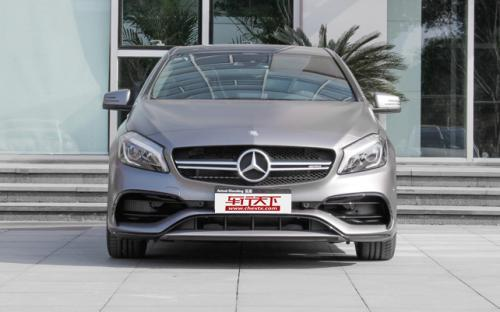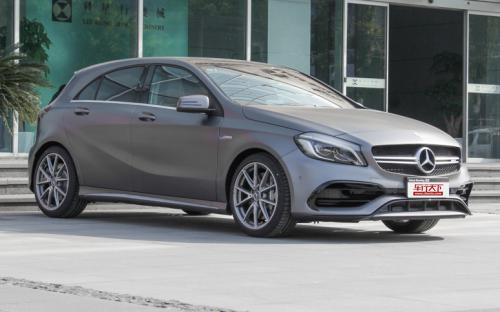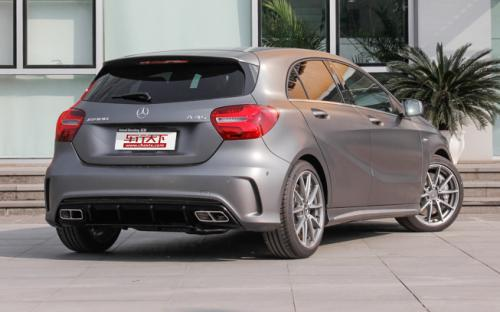### 奔驰AMG 奔驰A级AMG10 种颜色可选2016款最低售价：49.80 万元起

4367(mm)1780(mm)1417(mm)##### 配置亮点：
• 胎压监测装置

• ISOFIX儿童座椅接口

• 车身稳定控制(ESC/ESP/DSC等)

• 电动天窗

• 定速巡航

• 后倒车雷达

• 真皮座椅

• GPS导航系统

• 氙气大灯

• 后视镜加热

• 提交
2016款 A 45 AMG 4MATIC (275张)
• 2016款 A 45 AMG 4MATIC (275张)
• 2016款 A 45 AMG 4MATIC 冠军版 (5张)
• 奔驰AMG 奔驰A级AMG 绕车实拍• 奔驰AMG 奔驰A级AMG 在售车型

排量 车型 厂商指导价 本地最低报价 购车工具
2.0T
A 45 AMG 4MATIC 7挡双离合
49.80万
59.20万

奔驰AMG 奔驰A级AMG 经销商

查看更多 >>

### 奔驰AMG 奔驰A级AMG 动力加速

奔驰A级AMG 0-100公里加速时间分布在 4.2-4.6秒 属于 高性能级

动力级别 加速时间 车型
高性能级(2款)
4.2s
A 45 AMG 4MATICA 45 AMG 4MATIC 冠军版
4.6s
A 45 AMG 4MATIC

奔驰AMG 奔驰A级AMG 视频

奔驰AMG 奔驰A级AMG 新闻资讯

# 梅赛德斯AMG A 45冠军版上市 59.2万起

进口新车 超过8305次关注

日前，梅赛德斯-AMG正式宣布，A45冠军版车型正式上市，从外观上采用了和梅赛德斯-AMG车队W06Hybrid赛车颜色相同的马石油绿色拉花，使用了19英寸的多条辐钛色轮圈，...

改装 超过1次关注

# 运动是专长 实拍奔驰CLA45 AMG/A45 AMG

评测 超过4541次关注

说到AMG这三个字母，很多人首先能够联想到的便是运动，其次才是豪华，而如果在它前面加上CLA45及A45的话，仿佛有股热血马上就会涌上来。是的，这两部正是AMG系列中...

# 奔驰A45 AMG售49.8万 2014北京车展

新闻 超过5607次关注

在本届北京车展上，奔驰A45AMG车型售价正式公布，该车搭载的是2.0T高功率发动机，售价为49.8万元。此外，新车还将提供带有运动套件的车型可供选择，但具体价格官方...

# 奔驰AMG动力计划曝光 布局2.0T发动机

新闻 超过5772次关注

随着奔驰A45AMG和CLA45AMG等车型的亮相，拉开了奔驰AMG正式开始启用四缸涡轮增压发动机的序幕。日前，国外媒体报道，AMG未来将会更多的使用四缸涡轮增压发动机。

# A45 AMG Erika版官图 黑色/粉色双色调

新闻 超过5525次关注

日前，奔驰官方发布了A45AMGErika版车型，新车的外观和内饰都采用了专属的设计，十分个性。

改装 超过4668次关注

猜你喜欢

﻿
• 快速找车
• 选择品牌
• 选择品牌
• A  奥迪
• A  阿斯顿·马丁
• A  阿尔法·罗密欧
• B  宝沃
• B  布加迪
• B  巴博斯
• B  保时捷
• B  宾利
• B  奔驰
• B  宝马
• B  本田
• B  别克
• B  标致
• B  比亚迪
• B  宝骏
• B  北汽制造
• B  北汽新能源
• B  北汽幻速
• B  北汽威旺
• B  北京汽车
• B  奔腾
• B  北汽绅宝
• C  长安
• C  长安商用
• C  长城
• C  昌河
• D  大众
• D  道奇
• D  DS
• D  东南
• D  东风风神
• D  东风风行
• D  东风小康
• D  东风风度
• D  东风
• F  福特
• F  丰田
• F  菲亚特
• F  法拉利
• F  福田
• F  福迪
• F  福汽启腾
• G  观致
• G  广汽传祺
• G  广汽吉奥
• G  GMC
• H  红旗
• H  汉腾汽车
• H  哈弗
• H  哈飞
• H  海格
• H  海马
• H  华颂
• H  黄海
• H  华泰
• H  恒天
• J  吉利汽车
• J  捷豹
• J  Jeep
• J  江淮
• J  江铃
• J  金杯
• J  九龙
• J  金旅
• K  凯翼
• K  凯迪拉克
• K  克莱斯勒
• K  科尼塞克
• K  卡威
• K  开瑞
• L  路虎
• L  林肯
• L  劳斯莱斯
• L  兰博基尼
• L  雷克萨斯
• L  铃木
• L  雷诺
• L  理念
• L  力帆
• L  莲花汽车
• L  猎豹
• L  路特斯
• L  陆风
• M  马自达
• M  MG
• M  MINI
• M  玛莎拉蒂
• M  摩根
• M  迈凯轮
• N  纳智捷
• O  欧宝
• O  讴歌
• O  欧朗
• Q  奇瑞
• Q  起亚
• Q  启辰
• R  日产
• R  荣威
• R  瑞麒
• S  三菱
• S  斯威汽车
• S  萨博
• S  smart
• S  斯柯达
• S  斯巴鲁
• S  思铭
• S  双龙
• S  上汽大通
• S  双环
• T  特斯拉
• T  腾势
• W  沃尔沃
• W  五菱汽车
• W  五十铃
• W  威兹曼
• W  威麟
• X  现代
• X  雪佛兰
• X  雪铁龙
• X  西雅特
• Y  一汽
• Y  英菲尼迪
• Y  英致
• Y  依维柯
• Y  野马汽车
• Y  永源
• Z  众泰
• Z  中华
• Z  中兴
• Z  知豆
• 选择车系
• 选择车系
• 车型对比
• 选择品牌
• 选择品牌
• A  奥迪
• A  阿斯顿·马丁
• A  阿尔法·罗密欧
• B  宝沃
• B  布加迪
• B  巴博斯
• B  保时捷
• B  宾利
• B  奔驰
• B  宝马
• B  本田
• B  别克
• B  标致
• B  比亚迪
• B  宝骏
• B  北汽制造
• B  北汽新能源
• B  北汽幻速
• B  北汽威旺
• B  北京汽车
• B  奔腾
• B  北汽绅宝
• C  长安
• C  长安商用
• C  长城
• C  昌河
• D  大众
• D  道奇
• D  DS
• D  东南
• D  东风风神
• D  东风风行
• D  东风小康
• D  东风风度
• D  东风
• F  福特
• F  丰田
• F  菲亚特
• F  法拉利
• F  福田
• F  福迪
• F  福汽启腾
• G  观致
• G  广汽传祺
• G  广汽吉奥
• G  GMC
• H  红旗
• H  汉腾汽车
• H  哈弗
• H  哈飞
• H  海格
• H  海马
• H  华颂
• H  黄海
• H  华泰
• H  恒天
• J  吉利汽车
• J  捷豹
• J  Jeep
• J  江淮
• J  江铃
• J  金杯
• J  九龙
• J  金旅
• K  凯翼
• K  凯迪拉克
• K  克莱斯勒
• K  科尼塞克
• K  卡威
• K  开瑞
• L  路虎
• L  林肯
• L  劳斯莱斯
• L  兰博基尼
• L  雷克萨斯
• L  铃木
• L  雷诺
• L  理念
• L  力帆
• L  莲花汽车
• L  猎豹
• L  路特斯
• L  陆风
• M  马自达
• M  MG
• M  MINI
• M  玛莎拉蒂
• M  摩根
• M  迈凯轮
• N  纳智捷
• O  欧宝
• O  讴歌
• O  欧朗
• Q  奇瑞
• Q  起亚
• Q  启辰
• R  日产
• R  荣威
• R  瑞麒
• S  三菱
• S  斯威汽车
• S  萨博
• S  smart
• S  斯柯达
• S  斯巴鲁
• S  思铭
• S  双龙
• S  上汽大通
• S  双环
• T  特斯拉
• T  腾势
• W  沃尔沃
• W  五菱汽车
• W  五十铃
• W  威兹曼
• W  威麟
• X  现代
• X  雪佛兰
• X  雪铁龙
• X  西雅特
• Y  一汽
• Y  英菲尼迪
• Y  英致
• Y  依维柯
• Y  野马汽车
• Y  永源
• Z  众泰
• Z  中华
• Z  中兴
• Z  知豆
• 选择车系
• 选择车系
• 选择车型
• 选择车型
• 意见反馈